# Simply-connected group

(diff) ← Older revision | Latest revision (diff) | Newer revision → (diff)
Jump to: navigation, search

A topological group (in particular, a Lie group) for which the underlying topological space is simply-connected. The significance of simply-connected groups in the theory of Lie groups is explained by the following theorems.

1) Every connected Lie groupis isomorphic to the quotient group of a certain simply-connected group (called the universal covering of) by a discrete central subgroup isomorphic to.

2) Two simply-connected Lie groups are isomorphic if and only if their Lie algebras are isomorphic; furthermore, every homomorphism of the Lie algebra of a simply-connected group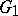into the Lie algebra of an arbitrary Lie group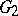is the derivation of a (uniquely defined) homomorphism ofinto.

The centreof a simply-connected semi-simple compact or complex Lie groupis finite. It is given in the following table for the various kinds of simple Lie groups.'
<tbody> </tbody>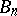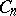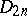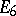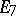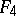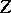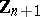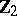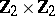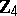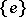In the theory of algebraic groups (cf. Algebraic group), a simply-connected group is a connected algebraic groupnot admitting any non-trivial isogeny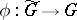, where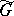is also a connected algebraic group. For semi-simple algebraic groups over the field of complex numbers this definition is equivalent to that given above.